# Partial derivative

of the first order of a function in several variables

The derivative of the function with respect to one of the variables, keeping the remaining variables fixed. For example, if a functionis defined in some neighbourhood of a point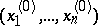, then the partial derivative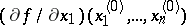ofwith respect to the variable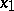at that point is equal to the ordinary derivative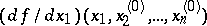at the pointof the function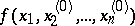in the single variable. In other words,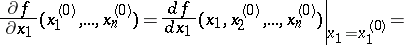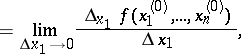where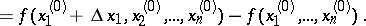The partial derivatives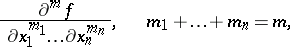(*)

of order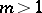are defined by induction: If the partial derivative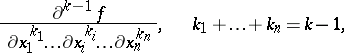has been defined, then by definition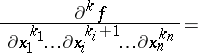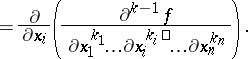The partial derivative (*) is also denoted by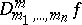. A partial derivative (*) in which at least two distinct indicesare non-zero is called a mixed partial derivative; otherwise, that is, if the partial derivative has the form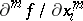, it is called unmixed. Under fairly broad conditions, mixed partial derivatives do not depend on the order of differentiation with respect to the different variables. This holds, for example, if all the partial derivatives under consideration are continuous.

If in the definition of a partial derivative the usual notion of a derivative is replaced by that of a generalized derivative in some sense or another, then the definition of a generalized partial derivative is obtained.